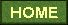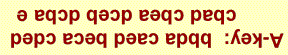#### DATA, CONSTANTS AND FORMULAS

 CONSTANTS  Avogadro    N = 6.022  ´ 1023 /mole speed of light   c = 2.998 ´ 108 m s-1 mass electron  me = 9.109 ´ 10-31 kg Planck   h = 6.626 ´ 10-34 J-s Bohr   ao = 52.92 pm Bohr   eo = 2.179 ´ 10-18 J  Rydberg   RH = 1.097 ´ 107 m-1 FORMULAS light wave:   ln = c photon energy:  E = hn HYDROGEN-LIKE ATOMS 1/l = RH Z2(1/n12-1/n22)   rn = ao n2/Z En = - eo Z2/n2 CONVERSION FACTORS 1 J = 1 kg  m2 s-2 1 cal = 4.184 J angstrom 1 Å = 10-10 m   1 nm = 10-9 m 1 pm = 10-12 m

## SOLUBLE IONIC COMPOUNDS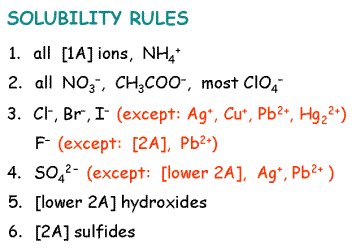STANDARD HEATS OF FORMATION

 C2H5OH( g) -235  kJ C2H5OH(l ) -278  kJ CH3CHO( g) -166  kJ CH3CHO(l ) -192  kJ CO( g) -110  kJ CO2( g) -394  kJ CO2(aq) -413  kJ H2O( g) -242  kJ H2O(l ) -286  kJ NH3( g) -46  kJ NH3(aq) -81 kJ NO2( g) 33 kJ

PERIODIC TABLE OF ELEMENTS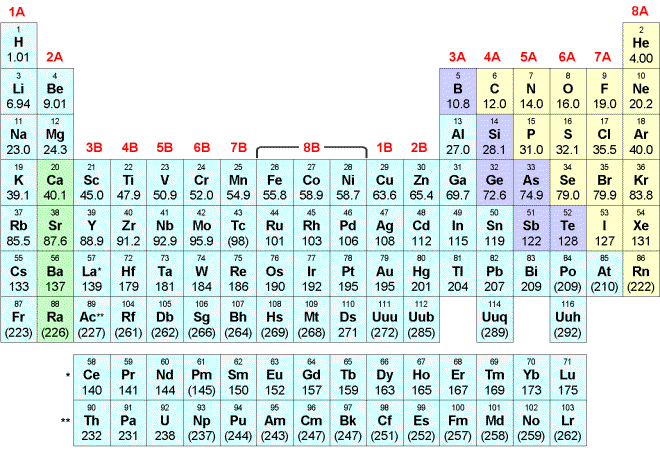Select one answer for each question:

1.   Which statement is TRUE?

 A) As the energy increases, the frequency of the radiation decreases. B) The product of wavelength and frequency of light is a constant. C) As the wavelength of the light increases, the frequency increases. D) Red light has a higher frequency than blue light. E) Light is considered to have only wave character.

2.  The electron configuration of Na is

 A) 1s2 2s2 2p6 3p B) 1s2 2s2 2p6 3s C) 1s2 2s2 2p3 3s D) 1s2 2s2 2p3 3d E) 1s2 2s2 2p3 3p

3.  When Ne is ionized the orbital from which the electron is removed has

 A) n = 1,  l = 0 B) n = 1, l = 1 C) n = 2,  l = 2 D) n = 2,  l = 1 E) n = 2,  l = 0

4.   In which atom does the electron configuration in the highest subshell look like this?A) Te B) As C) Sn D) Sb E) Cl

5.  How many orbitals are there in the n=4 level of the hydrogen atom?

 A) 14 B) 6 C) 16 D) 10 E) 20

6.  How many electrons can be filled into the 5d subshell?

 A) 10 B) 25 C) 15 D) 5 E) 12

7.   How many electrons in Xe occupy d orbitals with ml = +1?

 A) 10 B) 18 C) 2 D) 6 E) 4

8.  Which of these atoms has the smallest radius?

 Size trends of atoms other than hydrogen will not be on the CH221 final A) Xe B) Al C) Ca D) F E) N

9. According to ____ a particle of mass m and velocity v has a wavelength l = h/mv.

 A) Rutherford B) de Broglie C) Heisenberg D) Bohr E) Planck

10.  Which shape is consistent with a 3p orbital?

 A) B) C) D) E)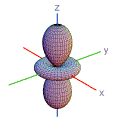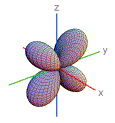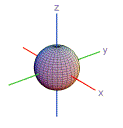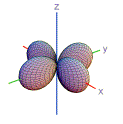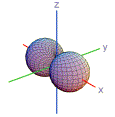11.  "Paramagnetic" atoms are attracted to a magnetic field because they contain unpaired electron spins.  Which atom is NOT paramagnetic?

 A) Cs B) P C) Mg D) Al E) O

12.  According to ________ the energy of a light wave in the photoelectric effect is the E = hn.

 A) Einstein B) Bohr C) Heisenberg D) Pauli E) Davison and Germer

13.   The best description of the atom reaction  Na + Cl  ®   Na+ + Cl- is:

 A) The electron jumps from the 3p orbital on Na to the 3p orbital on Cl. B) The electron jumps from the 3s orbital on Na to the 3s orbital on Cl. C) The electron jumps from the 3s orbital on Na to the 3p orbital on Cl. D) The electron jumps from the 3p orbital on Na to the 3s orbital on Cl.

14.   The ionization energy ("work function") of cesium is 6.24 ´ 10-19 J/atom.  Find the minimum  frequency of light required to ionize a cesium atom.

 A) 1.06 ´ 10-15 s-1 B) 9.42 ´ 1011 s-1 C) 1.06 ´ 10-12 s-1 D) 9.41 ´ 1014 s-1 E) 4.13 ´ 1014 s-1

15.  What is the wavelength (in nanometers) of light emitted when the hydrogen atom undergoes a transition from level n = 5 to level n = 2?

 A) 663 nm B) 833 nm C) 604 nm D) 546 nm E) 434 nm

16.  What is the ionization energy (in kJ/mole) of the hydrogen-like ion F8+

 A) 1.06 ´ 103 B) 1.31 ´ 104 C) 1.59 ´ 105 D) 1.06 ´ 105 E) 8.40 ´ 104

17.  The mass (in grams) of one N2O4 molecule is:

 A) 92.0 B) 46.0 C) 1.53 ´ 10-22 D) 1.15 ´ 10-22 E) 7.64 ´ 10-23

18.   When HCl reacts with NaOH in aqueous solution the net ionic equation is

 A) Na+(aq) + Cl- (aq)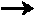NaCl (s) B) H+(aq) + OH-(aq)H2O(l) C) OH-(aq) + HCl(aq)H2O(l) + Cl- (aq) D) H+(aq) + NaOH(aq)Na+(aq) + H2O(l) E) no reaction

19.   What is the change in enthalpy (kJ) when 1 mole (28.0 g) of carbon monoxide is oxidized to carbon dioxide?

2 CO(g) + O2 (g)2 CO2 (g) + 566 kJ

 A) -566/2 B) -566 C) 566 / 2 D) 566 E) 566 ´ 2

20.   Which of these ionic compounds is least soluble in water?

 A) (NH4)2CO3 B) Pb(NO3)2 C) MgCl2 D) Ca3(PO4)2 E) NaCl

21.  Oxidation-reduction reactions involve transfer of

 A) electrons to the reducing agent from the oxidizing agent B) protons to the oxidizing agent from the reducing agent C) electrons to the oxidizing agent from the reducing agent D) protons to the reducing agent from the oxidizing agent

22.  The oxidation number of antimony in SbCl63-  is

 A) 5 B) 3 C) 6 D) 4 E) 2

23.   Balance the equation with lowest integer coefficients and give the coefficient of H+.

_Fe2+ + _ H+ +  _Cr2O72-_Cr3+ + _Fe3+ + _ H2O

 balancing redox equations will not be on the CH221 final A) 16 B) 18 C) 12 D) 10 E) 14

24.  The reactant that is reduced in the equation in Question 23 is:

 A) Cr2O72- B) H+ C) Fe2+ D) none--this is not a redox reaction

25.   Use these two reactions

2 Fe + 1.5 O2Fe2O3     DH = -823 kJ

3 Fe + 2 O2Fe3O4 ,       DH = -1120 kJ

to find the enthalpy change for the reaction

3 Fe2O32 Fe3O4 +  0.5 O2

 A) 256 kJ B) -229 kJ C) 245 kJ D) 229 kJ E) -256 kJ

26.  A chemical system at constant pressure absorbs 50 kJ of heat from the surroundings.  The enthalpy change of the system is best described as:

 A) DH = -50 kJ (endothermic) B) DH =  50 kJ (exothermic) C) DH =  50 kJ (endothermic) D) DH = -50 kJ (exothermic)

27.  How much heat is lost when 35.5 g of iron cools from 429°C  to 18.6°C ?   The specific heat of iron is 0.450 J/g-°C.

 A) 14,600 J B) 32,400 J C) 4820 J D) 8240 J E) 6560 J

28.   Find the moles of electrons transferred in the balanced oxidation-reduction reaction

C6H12O6  + 6 O26 CO2  +  6 H2O

 this type of redox problem will not be on the CH221 final A) 16 B) 24 C) 48 D) 36 E) 12

29.  How many moles of atoms are there in one mole of iron (III) sulfate?

 A) 18 B) 16 C) 15 D) 17 E) 20

30.  The empirical formula of a compound SbxOy that contains 75.31% antimony (Sb) by  weight is

 A) SbO2 B) Sb2O3 C) Sb2O5 D) SbO4 E) SbO3

31.  How many grams of sulfate ions are in 0.200 L of 0.0300 M  Al2(SO4)3  solution?

 A) 2.88 B) 1.73 C) 8.06 D) 1.15 E) 2.73

32.  How many mL of 0.080 M sulfuric acid (H2SO4, MW = 98.1) are required for complete neutralization of 40.0 mL of  0.050 M aluminum hydroxide (Al(OH)3, MW = 78.0)?

 A) 37.5 B) 16.7 C) 51.4 D) 62.8 E) 75.1

33.  What is the theoretical mass yield of tetraphosphorous hex(a)oxide produced by combustion of 8.00 g of phosphorous in excess oxygen?

 A) 42.6 g B) 227. g C) 56.8 g D) 28.4 g E) 14.2 g